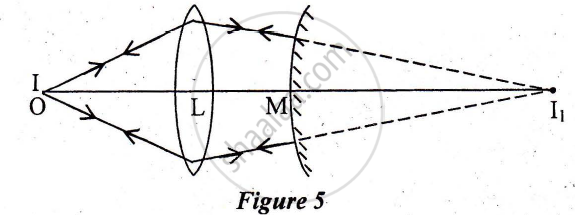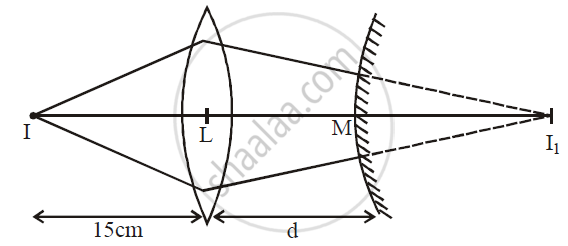# A Point Object O is Placed at a Distance of 15cm from a Convex Lens L of Focal Length 1 Ocm as Shown in Figure 5 Below. on the Other Side of the Lens, a Convex Mirror M is Placed Such that Its Distance from the Lens is Equal to the Focal Length of the Lens. the Final Image Formed by this Combination is Observed to Coincide with the Object O. Find the Focal Length of the Convex Mirror - Physics (Theory)

A point object O is placed at a distance of 15cm from a convex lens L of focal length 1 Ocm as shown in Figure 5 below. On the other side of the lens, a convex mirror M is placed such that its distance from the lens is equal to the focal length of the lens. The final image formed by this combination is observed to coincide with the object O. Find the focal length of the convex mirror#### Solutionu = 15 cm

f = 10 cm

d = f = 10 cm

For lens, 1/V - 1/u = 1/f

1/V - 1/((-15)) = 1/10

1/V = 1/10 -= 1/15 = (3-2)/30 = 1/30

V= 30 cm

MI = LI - LM = 30 - 10 = 20 cm

f = 20/2 = 10 cm

Is there an error in this question or solution?
2014-2015 (March)

Share